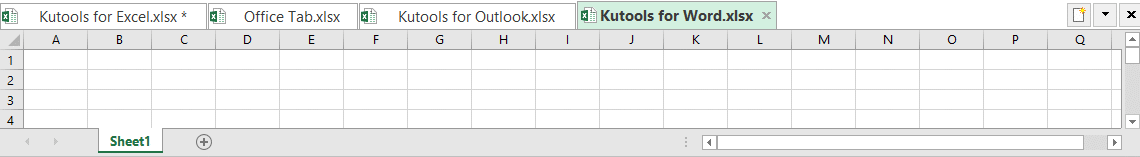## Average data in multiple non-contiguous ranges in Excel

In Excel, you can use the function AVERAGE to calculate the average value in a selected range. But sometimes, the values are in non-contiguous ranges which needed to be calculated, in this case, how can you quickly average these values in multiple ranges including zero or excluding zero in Excel.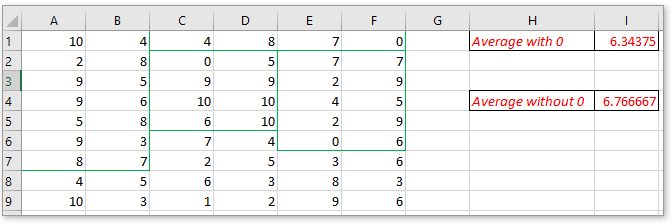Average data in multiple non-contiguous ranges including zero

Average data in multiple non-contiguous ranges excluding zero

#### Average data in multiple non-contiguous ranges including zero

If you want to average data in multiple range which are non-contiguous, supposing, you want to average the ranges A1:B7, C2:D5 and E2:F6 as below screenshot shown, you can do as below steps: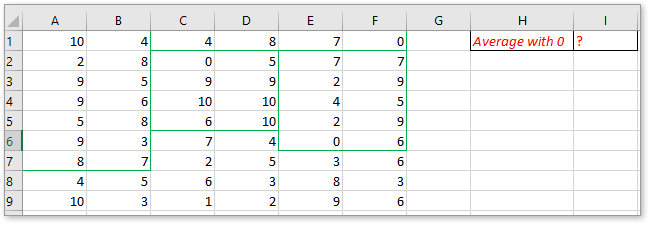1. Add the ranges one by one into the AVERAGE function.

Type or copy this formula:

=AVERAGE(A1:B7,C2:D5,E2:F6)

Using commas to separate the non-contiguous ranges in the formula, you can add as many ranges as you need.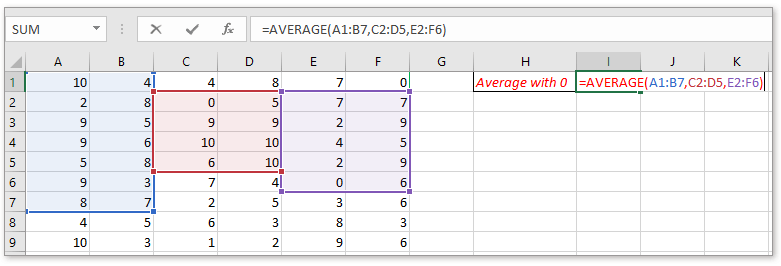Press Enter key.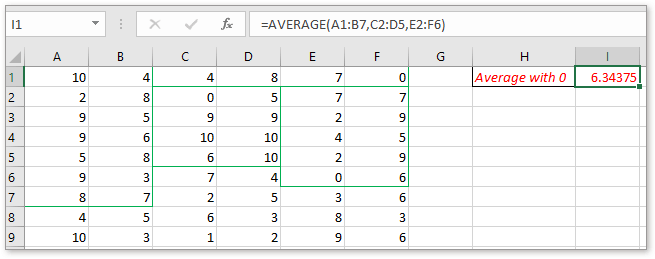2. Define a range name to non-contiguous ranges

If you do not want to add multiple range references to the AVERAGE function, you can give a range name to the ranges you want to calculate firstly. Holding Ctrl key to select the ranges you want to average, then go to the Name box (at the left of formula bar), type a name, then press Enter key to define. See screenshot: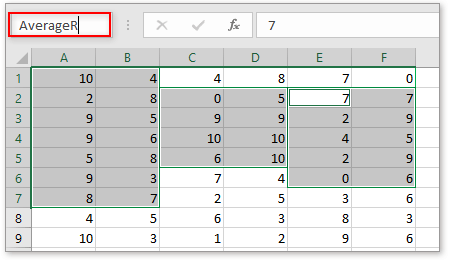Then type this formula in a cell

=AVERAGE(AverageR)

Press Enter key.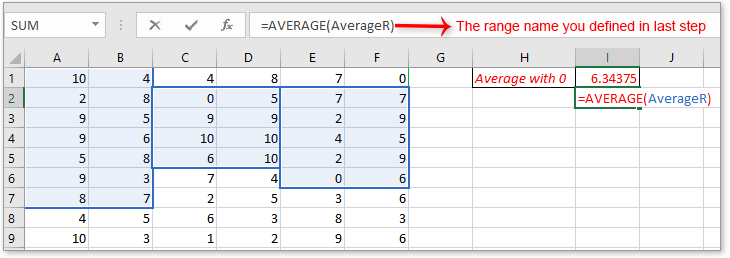Tip: If you are in trouble with remembering complex formulas, here the Auto Text tool of Kutools for Excel can save all formulas you used in a pane for you, then, you can reuse them in anywhere anytime, what you only need to do is change the references to match your real need.  Click for free download it now.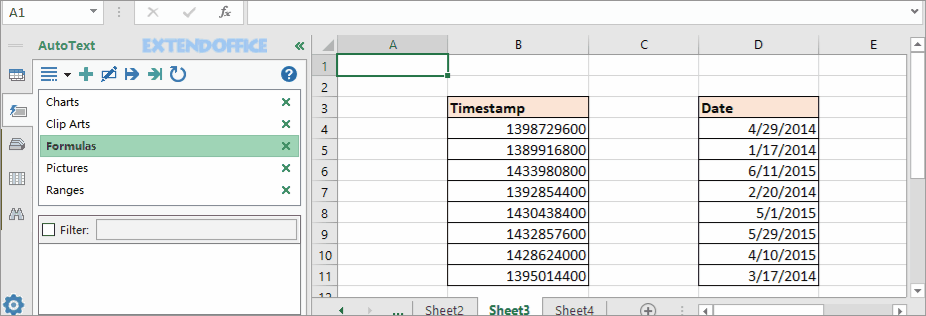#### Average data in multiple non-contiguous ranges excluding zero

If you want to average the values in non-contiguous ranges excluding 0, you can use below formula:

=SUM(A1:B7,C2:D5,E2:F6)/INDEX(FREQUENCY((A1:B7,C2:D5,E2:F6),0),2)

Press Enter key.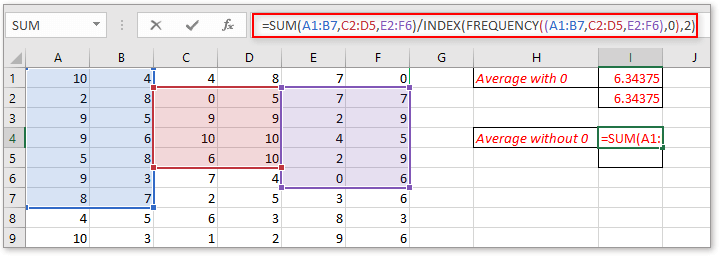Also, you can define the ranges a range name, then use the formula

=SUM(AverageR)/INDEX(FREQUENCY((AverageR),0),2)

AverageR is the range name you defined for the range A1:B7, C2:D5 and E2:F6. Press Enter key.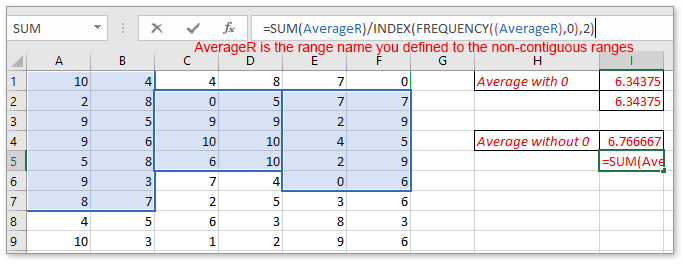Note: Both formulas introduced in this section are not fit to calculate averages of ranges which include negative values.

#### Other Operations (Articles) Related To Calculation

Count/sum the cells greater than but less than a number
In this articl, it provides the methods on count or sum cells which are greater than or less than a specified number.

Sum every other or nth row/column in Excel
As we all know, we can apply the Sum function to add a list of cells, but sometimes we need to sum every other cell for some purpose, and Excel has no standard function allow us to sum every nth cell. In this situation, how could we sum every other or nth row / column in Excel?

Summarize data from worksheets / workbooks into one worksheet
Supposing you have a workbook which contains multiple worksheets, and each worksheet has the identical layout. And now you need to merge the multiple worksheets and calculate the final results into a master worksheet, how could you consolidate or summarize data from multiple worksheets or workbooks into a master worksheet?

Average based on day of week in Excel
In Excel, have you ever tried to calculate the average of a list number depending on which day of the week?

## The Best Office Productivity Tools

### Kutools for Excel Solves Most of Your Problems, and Increases Your Productivity by 80%

• Super Formula Bar (easily edit multiple lines of text and formula); Reading Layout (easily read and edit large numbers of cells); Paste to Filtered Range...
• Merge Cells/Rows/Columns and Keeping Data; Split Cells Content; Combine Duplicate Rows and Sum/Average... Prevent Duplicate Cells; Compare Ranges...
• Select Duplicate or Unique Rows; Select Blank Rows (all cells are empty); Super Find and Fuzzy Find in Many Workbooks; Random Select...
• Exact Copy Multiple Cells without changing formula reference; Auto Create References to Multiple Sheets; Insert Bullets, Check Boxes and more...
• Favorite and Quickly Insert Formulas, Ranges, Charts and Pictures; Encrypt Cells with password; Create Mailing List and send emails...
• Extract Text, Add Text, Remove by Position, Remove Space; Create and Print Paging Subtotals; Convert Between Cells Content and Comments...
• Super Filter (save and apply filter schemes to other sheets); Advanced Sort by month/week/day, frequency and more; Special Filter by bold, italic...
• Combine Workbooks and WorkSheets; Merge Tables based on key columns; Split Data into Multiple Sheets; Batch Convert xls, xlsx and PDF...
• Pivot Table Grouping by week number, day of week and more... Show Unlocked, Locked Cells by different colors; Highlight Cells That Have Formula/Name...### Office Tab - brings tabbed interface to Office, and make your work much easier

• Enable tabbed editing and reading in Word, Excel, PowerPoint, Publisher, Access, Visio and Project.
• Open and create multiple documents in new tabs of the same window, rather than in new windows.
• Increases your productivity by 50%, and reduces hundreds of mouse clicks for you every day!